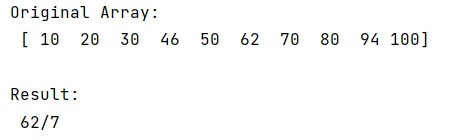# Is there any function to reduce fractions?

Learn, whether there is any function to reduce fractions in Python?
Submitted by Pranit Sharma, on February 21, 2023

NumPy is an abbreviated form of Numerical Python. It is used for different types of scientific operations in python. Numpy is a vast library in python which is used for almost every kind of scientific or mathematical operation. It is itself an array which is a collection of various methods and functions for processing the arrays.

## Function to reduce fractions

Here, we'll how to reduce fractions in p/q form rather than find the exact answer. For example, if we need to get the result of 98/42 then we need 7/3 instead of 2.33333.

Suppose that we are given a numpy array and we need to divide the elements of this numpy array with a specific number we do not want the result as a float value but just another fraction in the form of p/q.

For this purpose, we have the fraction() method from the fractions module. We will simply use Fractions where we will pass the two numbers (numerator and denominator) and it will result in a reduced fraction.

Let us understand with the help of an example,

## Python code to demonstrate that is there any function to reduce fractions

```# Import numpy
import numpy as np

# Importing Fraction
from fractions import Fraction

# Creating a numpy array
arr = np.array([10,20,30,46,50,62,70,80,94,100])

# Display original array
print("Original Array:\n",arr,"\n")

# Dividing the numpy array with 7
res = Fraction(arr,7)

# Display result
print("Result:\n",res)
```

Output: